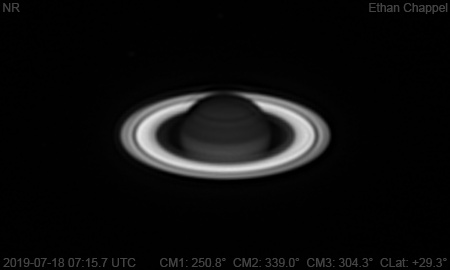# Saturn 2019-07-18 07:15 UTC

CM1: 250.80°

CM2: 339.00°

CM3: 304.30°

CLat: +29.30°

Description

An excellent night for planetary imaging, with the usual caveat of being too breezy for using the CGEM DX/C14.

Notes:

• The EZ is split by a thin band, which is barely visible in visible light and near-infrared.
• Enceledus is just below the 9 o'clock position relative to Saturn, Dione is at 12 o'clock, and Tethys is between the two aforementioned moons, slightly closer to Dione.

Equipment

ZWO ASI290MM

Celestron EdgeHD 8

Logs
```FireCapture v2.6  Settings
------------------------------------
Observer=Ethan Chappel
Camera=ZWO ASI290MM
Filter=CH4
Profile=Saturn
Diameter=18.30"
Magnitude=0.10
CMI=252.2° CMIII=305.7°  (during mid of capture)
FocalLength=1950mm (F/6)
Resolution=0.31"
Filename=2019-07-18-0718_2-EC-CH4-Sat.ser
Date=2019_07_18
Start=07_15_46.138
Mid=07_18_16.146
End=07_20_46.155
Start(UT)=07_15_46.138
Mid(UT)=07_18_16.146
End(UT)=07_20_46.155
Duration=300.017s
Date_format=yyyy_MM_dd
Time_format=HH_mm_ss
LT=UT -6h
Frames captured=4616
File type=SER
Binning=no
Bit depth=8bit
Debayer=no
ROI=412x246
ROI(Offset)=0x0
FPS (avg.)=15
Shutter=65.00ms
Gain=420 (70%)
Gamma=50
SoftwareGain=10 (off)
AutoExposure=off
Brightness=1 (off)
USBTraffic=40 (off)
AutoHisto=75 (off)
HighSpeed=off
FPS=100 (off)
AutoGain=off
Histogramm(min)=0
Histogramm(max)=243
Histogramm=95%
Noise(avg.deviation)=2.50
AutoAlign=false
PreFilter=none
Limit=300 Seconds
Sensor temperature=30.8°C
FireCapture v2.6  Settings
------------------------------------
Observer=Ethan Chappel
Camera=ZWO ASI290MM
Filter=CH4
Profile=Saturn
Diameter=18.30"
Magnitude=0.10
CMI=249.3° CMIII=302.8°  (during mid of capture)
FocalLength=1950mm (F/6)
Resolution=0.31"
Filename=2019-07-18-0713_2-EC-CH4-Sat.ser
Date=2019_07_18
Start=07_10_42.699
Mid=07_13_12.703
End=07_15_42.707
Start(UT)=07_10_42.699
Mid(UT)=07_13_12.703
End(UT)=07_15_42.707
Duration=300.008s
Date_format=yyyy_MM_dd
Time_format=HH_mm_ss
LT=UT -6h
Frames captured=4616
File type=SER
Binning=no
Bit depth=8bit
Debayer=no
ROI=412x246
ROI(Offset)=0x0
FPS (avg.)=15
Shutter=65.00ms
Gain=420 (70%)
Gamma=50
SoftwareGain=10 (off)
AutoExposure=off
Brightness=1 (off)
USBTraffic=40 (off)
AutoHisto=75 (off)
HighSpeed=off
FPS=100 (off)
AutoGain=off
Histogramm(min)=0
Histogramm(max)=214
Histogramm=83%
Noise(avg.deviation)=2.50
AutoAlign=false
PreFilter=none
Limit=300 Seconds
Sensor temperature=31.1°C
```